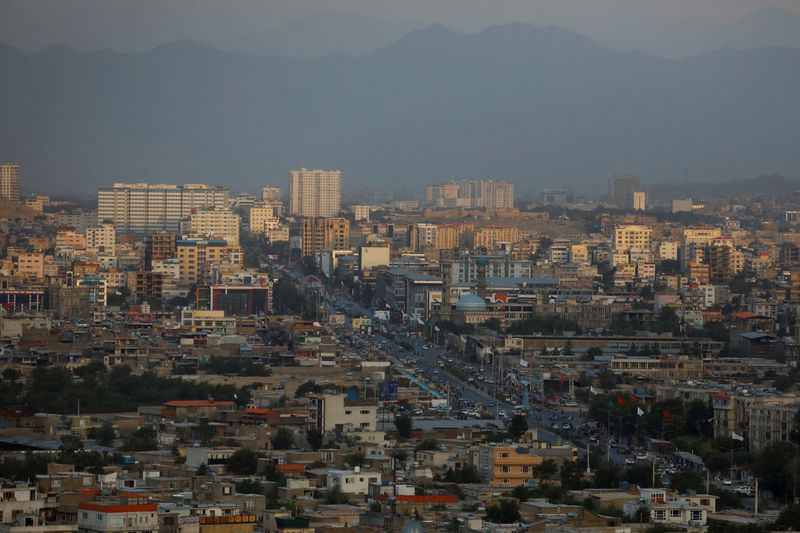# Volatility and Risk: What’s the Relationship?

Volatility and risk are two terms that are often used interchangeably, but there is a distinction between the two. In this blog post, we will explore the relationship between volatility and risk, and discuss why it is important for investors to understand both concepts. Volatility is a measure of how much prices change over time, while risk is a measure of how likely it is that you will lose money on an investment. Many people mistakenly believe that high volatility leads to high risk, but this is not always the case. In order to make informed investment decisions, it is essential to understand the difference between these two concepts.

## Are volatility and risk related?

Volatility is a measure of how much prices change over time. The most common way to measure volatility is by calculating the standard deviation of returns. Standard deviation is a statistical measure that quantifies how much variation there is in a set of data. A higher standard deviation indicates more volatility, while a lower standard deviation indicates less volatility.

Risk is a measure of how likely it is that you will lose money on an investment. Risk can be measured in a number of ways, but the most common method is to calculate the probability of a loss. Probability is a statistical measure that quantifies the likelihood of an event occurring. A higher probability indicates more risk, while a lower probability indicates less risk.

So, what is the relationship between volatility and risk? The answer is that they are not directly related. Volatility is a measure of price movements, while risk is a measure of the likelihood of losing money. Just because an investment has high volatility does not mean it is automatically high risk. Likewise, just because an investment has low volatility does not mean it is automatically low risk. It is important to consider both concepts when making investment decisions.

## Factors that impact the relationship between volatility and risk

There are a number of factors that can impact the relationship between volatility and risk. One of the most important is the time horizon. In general, the longer you hold an investment, the lower the risk. This is because there is more time for the price to recover from temporary dips. For example, a stock may be very volatile in the short term, but if you plan to hold it for several years, the risk is lower. This is one of the reasons why it is important to consider your time horizon when investing.

Another factor that can impact the relationship between volatility and risk is diversification. Diversification is the process of spreading your investment across a number of different asset classes. This can help to reduce risk because it ensures that you are not putting all of your eggs in one basket. For example, if you invest in a portfolio of stocks, bonds, and cash, you will be less exposed to the volatility of the stock market.

As you can see, there is a complex relationship between volatility and risk. Volatility is a measure of price movements, while risk is a measure of the likelihood of losing money. The two concepts are not directly related, but there are a number of factors that can impact their relationship. It is important to consider both concepts when making investment decisions.

## Closing thoughts

Volatility and risk are two important concepts that every investor should understand. By understanding the relationship between these two concepts, you will be better equipped to make informed investment decisions.

What do you think? Are volatility and risk related? Let us know in the comments below.

## Further questions

Have an answer to the questions below? Post it here or in the forum

Views
Question
70
views
136
views
LATEST NEWSLATEST NEWS